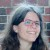# Given1. A pair of dice is rolled. What is theprobability of getting:a.) A sum less than 5?b.) the number o

Given
1. A pair of dice is rolled. What is the
probability of getting:
a.) A sum less than 5?
b.) the number of dots on both dice are
equal?

1.